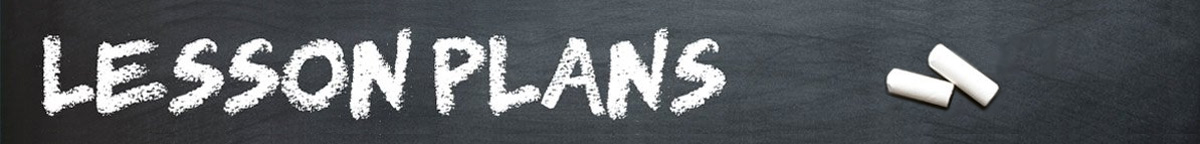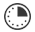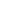# Box Geometry

JRDAssist
Middle School and High School Teacher

Students will learn about using the formulas for the area of squares, rectangles, and triangles to determine how much paint and carpet to purchase for a room.  In addition, they will learn about scale measurements.

##Length of Time: 40-50 Minutes

Featured Programs:

### Common Core Alignment

CCSS.Math.Content.7.G.B.6 - Solve real-world and mathematical problems involving area, volume and surface area of two- and three-dimensional objects composed of triangles, quadrilaterals, polygons, cubes, and right prisms.

## Objectives & Outcomes

The students will be able to use the formulas for the area of squares, rectangles, and triangles for real world applications.

## Materials Needed

• Various sizes of boxes
• scissors
• rulers
• paper
• pencil

## Procedure

### Opening to Lesson

• Once students are seated, the teacher will ask the class what color they would paint the walls of the room.  Accept several answers.
• Next, the teacher will ask the students what type of carpet they would like to have in the room.
• The teacher will then ask students what they estimate it would cost.
• Ask the students the question, “How do we determine how much paint and carpet would be needed?”
• After accepting the various answers, guide students to the correct method to determine the cost of painting and carpeting the room.

### Body of Lesson

#### Modeling

• Using the actual measurements of the classroom, help show students what they will be doing with their own rooms (boxes).
• Explain the procedure of subtracting windows and doorways from the total area of walls.
• Discuss the different costs of paints and carpets, per square foot or yard for coverage purposes.

#### Guided Practice

• Distribute a different size box to each student.  Have them cut two or three “windows” and “doors” in each box.
• Students will then measure the windows and doors, using a set scale to turn the inches or centimeters into feet or meters to approximate the size of a room.
• Students will measure the sizes of the “rooms”- boxes.
• Students will subtract doorway and window sizes from total surface area of the “walls”.
• Student will measure the “floor” of the room.
• The students will determine the amount of paint needed for the walls and the amount of carpet need for the floor.
• Using the advertisements, the students will estimate the cost of painting/carpeting the room.

#### Independent Practice

• The teacher will distribute a handout with several similar word problems and/or diagrams or blueprints of actual rooms for students to calculate the costs
• Assign students to calculate the cost of remodeling a room at their home
• The teacher will collect the completed page(s).  This will be used as an assessment.

### Closing

Ask students what other costs are involved in remodeling the room, such as labor, supplies, etc.  Have them think about the variables involved, such as extra coats of paint, plusher or more expensive carpeting.  Don’t forget the ceilings.

## Assessment & Evaluation

Handouts/Worksheets with word problems having similar questions related to estimating the amount of carpet and paint needed for rooms in a house or building.

## Modification & Differentiation

Students may work in pairs.  Have students switch their box with another student for further practice.  As a homework assignment or extra credit, have students measure an actual room in their home to determine amount of paint/carpet needed.  Have students determine the total cost of paint and carpet.

## Related Lesson Plans

##### Interest-ing Math

Students will learn about simple interest and how to calculate the real cost of a loan, credit card, and other types of borrowing.

##### The Ideal Town

Based on research the students will design a small town with a pre-determined population, assuring there is enough living space, parking spaces, and a sufficient water supply.

##### Silent Geometry

The students will practice using geometry formulas measuring items in the classroom to find area and volume, radius and circumference, and identify the types of angles.

##### Ice Cream Cones, Baseballs, and Cans

Students will work in pairs to practice finding the volume of cones, cylinders, and spheres using everyday objects.

##